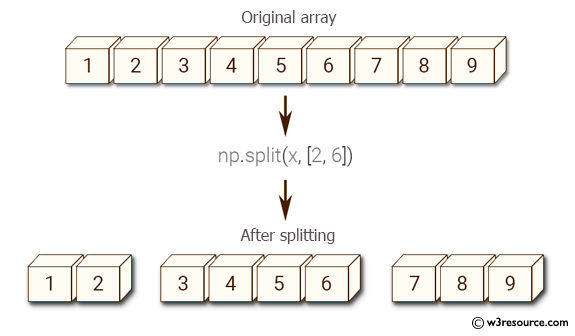﻿ NumPy: Split an array of 14 elements into 3 arrays - w3resource# NumPy: Split an array of 14 elements into 3 arrays

## NumPy: Array Object Exercise-61 with Solution

Write a Numpy program to split an array of 14 elements into 3 arrays, each of which has 2, 4, and 8 elements in the original order.
Sample array: [ 1 2 3 4 5 6 7 8 9 10 11 12 13 14]

Pictorial Presentation:Sample Solution:-

Python Code:

``````import numpy as np
x = np.arange(1, 15)
print("Original array:",x)
print("After splitting:")
print(np.split(x, [2, 6]))
```
```

Sample Output:

```Original array: [ 1  2  3  4  5  6  7  8  9 10 11 12 13 14]
After splitting:
[array([1, 2]), array([3, 4, 5, 6]), array([ 7,  8,  9, 10, 11, 12, 13,
14])]
```

Python Code Editor:

Have another way to solve this solution? Contribute your code (and comments) through Disqus.

What is the difficulty level of this exercise?

Test your Python skills with w3resource's quiz

﻿

## Python: Tips of the Day

Set comprehension:

```>>> m = {x ** 2 for x in range(5)}
>>> m
{0, 1, 4, 9, 16}
```